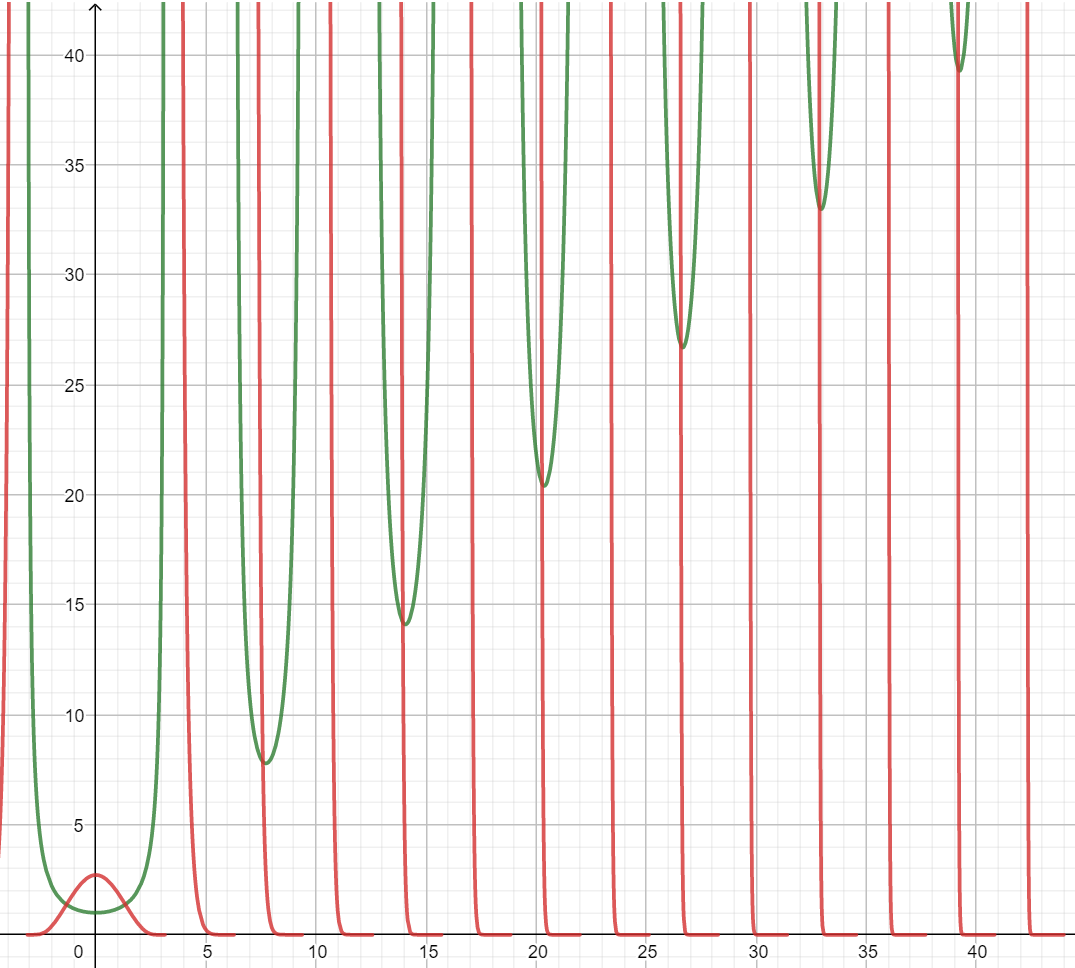# Constant Exponent

The equation in question is: ${ e }^{ x }=x$. It would be interesting to find a constant point in a function like exponent. Obviously there are no real solutions. But what if we consider complex numbers, are there any constant exponents?

We are looking for a complex number $z$ such as ${ e }^{ z }=z$. Let $z=x+iy$, where $x$ - real part, $y$ - imaginary part. Following the expanded definition of the exponent:

${ e }^{ x+iy }={ e }^{ x }\cdot (cos(y)+i\cdot sin(y))=x+iy$

Thus we get a system of two equations:

$\begin{cases} { x=e }^{ x }\cdot cos(y) \\ y={ e }^{ x }\cdot sin(y) \end{cases}$

Since there are no real solutions, $y\neq 0$ which also means $sin(y)\neq 0$. That means we can safely divide by $y$ and express $x$:

$\frac { x }{ y } =\frac { cos(y) }{ sin(y) }$

$x=y\cdot cot(y)$

Using this expression we get an equation that should help us get the value of $y$:

$y={ e }^{ y\cdot cot(y) }\cdot sin(y)$

Unfortunately, I wasn't able to derive a concrete root of this equation. However, let's consider these two functions:

$f(y)=\frac { y }{ sin(y) } \\ g(y)={ e }^{ y\cdot cot(y) }$

Both are even functions. If we build their graphs we get:($f(y)$ - green, $g(y)$ - red)

As we can see there is an infinite number of intersections occurring roughly once in every $2\pi$ interval. That means there is an infinite number of fixed complex points who are equal to their exponent which I find fascinating.

If you have any idea how to derive $z$ or found an error, feel free to comment.Note by Nick Kent
10 months ago

This discussion board is a place to discuss our Daily Challenges and the math and science related to those challenges. Explanations are more than just a solution — they should explain the steps and thinking strategies that you used to obtain the solution. Comments should further the discussion of math and science.

When posting on Brilliant:

• Use the emojis to react to an explanation, whether you're congratulating a job well done , or just really confused .
• Ask specific questions about the challenge or the steps in somebody's explanation. Well-posed questions can add a lot to the discussion, but posting "I don't understand!" doesn't help anyone.
• Try to contribute something new to the discussion, whether it is an extension, generalization or other idea related to the challenge.

MarkdownAppears as
*italics* or _italics_ italics
**bold** or __bold__ bold
- bulleted- list
• bulleted
• list
1. numbered2. list
1. numbered
2. list
Note: you must add a full line of space before and after lists for them to show up correctly
paragraph 1paragraph 2

paragraph 1

paragraph 2

[example link](https://brilliant.org)example link
> This is a quote
This is a quote
    # I indented these lines
# 4 spaces, and now they show
# up as a code block.

print "hello world"
# I indented these lines
# 4 spaces, and now they show
# up as a code block.

print "hello world"
MathAppears as
Remember to wrap math in $$ ... $$ or $ ... $ to ensure proper formatting.
2 \times 3 $2 \times 3$
2^{34} $2^{34}$
a_{i-1} $a_{i-1}$
\frac{2}{3} $\frac{2}{3}$
\sqrt{2} $\sqrt{2}$
\sum_{i=1}^3 $\sum_{i=1}^3$
\sin \theta $\sin \theta$
\boxed{123} $\boxed{123}$

Sort by:

@Nick Kent Would you like to post this as a problem?

- 10 months ago

Interesting investigation. Starting with your system of two equations, I used multi-variate Newton Raphson iteration to find $x$ and $y$.
Here is the $z$ value with the smallest magnitude. Of course, there are other solutions as well:

$z \approx 0.3181 \pm 1.3372 i$

Python code is attached:

  1 2 3 4 5 6 7 8 9 10 11 12 13 14 15 16 17 18 19 20 21 22 23 24 25 26 27 28 29 30 31 32 33 34 35 36 37 38 39 40 41 42 43 import math import numpy as np import random S = 5.0 # Initial guesses x = -S + 2.0*S*random.random() y = -S + 2.0*S*random.random() # Iterative solution for j in range(0,100): f1 = math.exp(x) * math.cos(y) - x # functions from system f2 = math.exp(x) * math.sin(y) - y fvec = np.array([f1,f2]) J11 = math.exp(x) * math.cos(y) - 1.0 # Jacobian matrix entries J12 = -math.exp(x) * math.sin(y) J21 = math.exp(x) * math.sin(y) J22 = math.exp(x) * math.cos(y) - 1.0 J = np.array([[J11,J12],[J21,J22]]) vec = np.array([x,y]) right = np.dot(J,vec) - fvec Sol = np.linalg.solve(J, right) x = Sol y = Sol print x print y print "" print "" print "###############################" print "" 

- 10 months ago